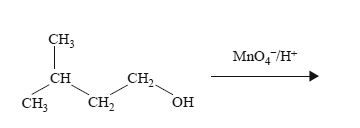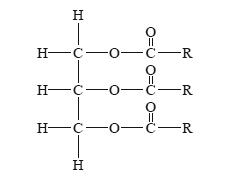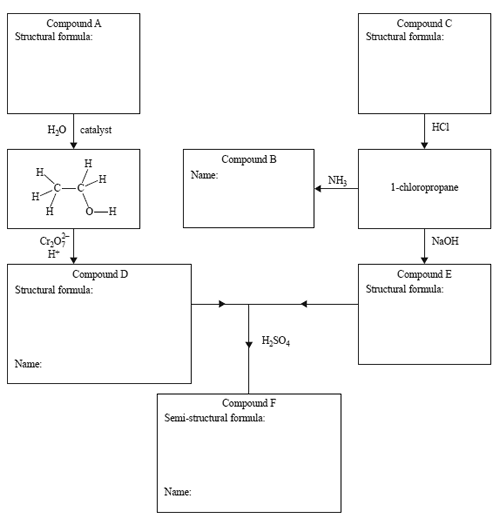Organic (2014 VCE) 1)What is the systematic name for the product of the reaction above? A. 2-methylpentanoic acid B. 4-methylpentanoic acid C. 2-methylbutanoic acid D. 3-methylbutanoic acid Solution2) The general formula of a triglyceride can be represented as follows.Which one of the following equations represents the hydrolysis of a triglyceride?Solution3) Compounds B and F may be synthesised as follows.a) Draw the structural formulae for Compounds A, C, D and E in the boxes provided. Solutionb) What are the systematic names of Compounds B and D? Solutionc) GIve the semi-structural formula and systematic name of Compound F Solution